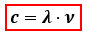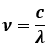# Problem: What wavelength of light will be required to remove an electron from the n = 3 shell of a hydrogen atom?

###### FREE Expert Solution
80% (117 ratings)
###### FREE Expert Solution

The wavelength of the light required to remove the electron from the n = 3 shell can be calculated using the speed of light:c = speed of light = 3.0x108 m/s
λ = wavelength, in m

Since we don’t have frequency, we can rearrange the equation:Recall the energy equation:

80% (117 ratings)###### Problem Details

What wavelength of light will be required to remove an electron from the n = 3 shell of a hydrogen atom?

What scientific concept do you need to know in order to solve this problem?

Our tutors have indicated that to solve this problem you will need to apply the Bohr and Balmer Equations concept. If you need more Bohr and Balmer Equations practice, you can also practice Bohr and Balmer Equations practice problems.

What is the difficulty of this problem?

Our tutors rated the difficulty ofWhat wavelength of light will be required to remove an elect...as medium difficulty.

How long does this problem take to solve?

Our expert Chemistry tutor, Sabrina took 5 minutes and 46 seconds to solve this problem. You can follow their steps in the video explanation above.

What professor is this problem relevant for?

Based on our data, we think this problem is relevant for Professor Bojan's class at PSU.